Anzeige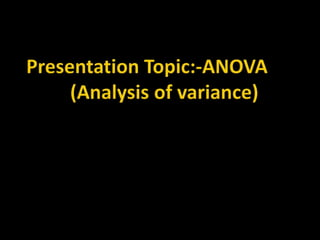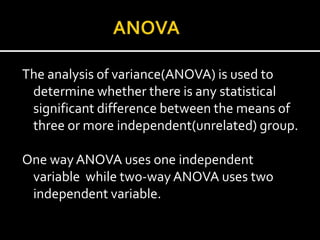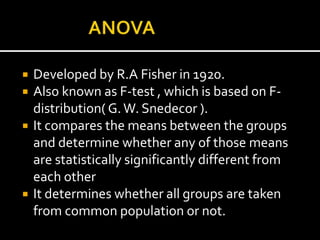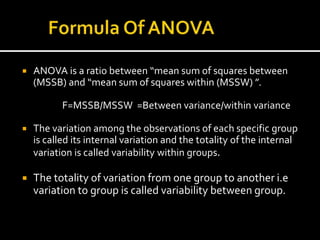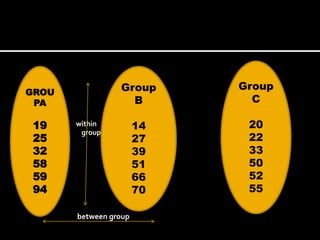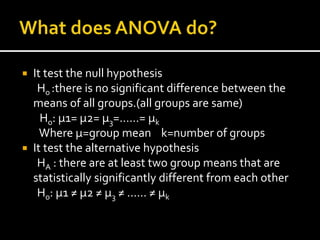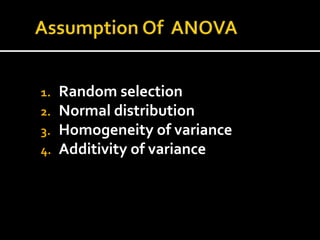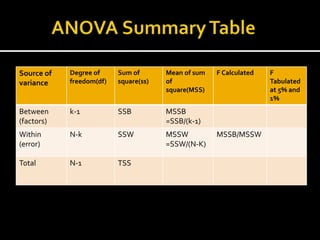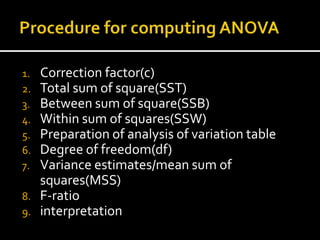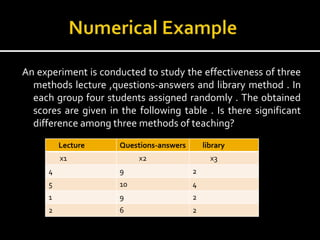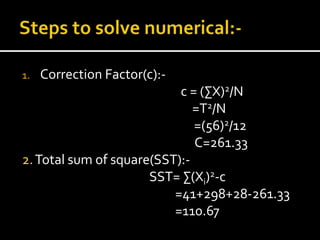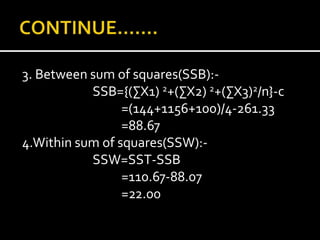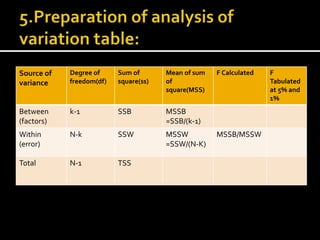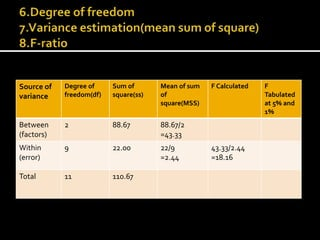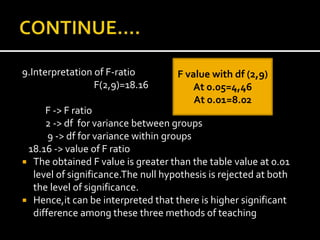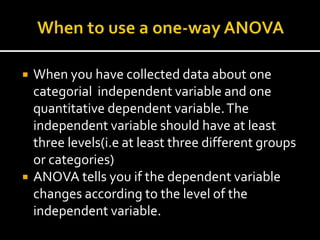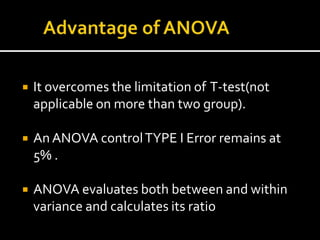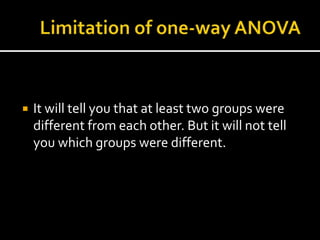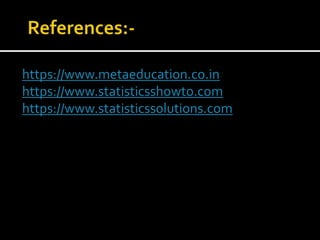1 von 20
Anzeige

### analysisofvariance-210906094053.pdf

1. The analysis of variance(ANOVA) is used to determine whether there is any statistical significant difference between the means of three or more independent(unrelated) group. One wayANOVA uses one independent variable while two-wayANOVA uses two independent variable.
2.  Developed by R.A Fisher in 1920.  Also known as F-test , which is based on F- distribution( G.W. Snedecor ).  It compares the means between the groups and determine whether any of those means are statistically significantly different from each other  It determines whether all groups are taken from common population or not.
3.  ANOVA is a ratio between “mean sum of squares between (MSSB) and “mean sum of squares within (MSSW) ”. F=MSSB/MSSW =Between variance/within variance  The variation among the observations of each specific group is called its internal variation and the totality of the internal variation is called variability within groups.  The totality of variation from one group to another i.e variation to group is called variability between group.
4.  within  group between group GROU PA 19 25 32 58 59 94 Group B 14 27 39 51 66 70 Group C 20 22 33 50 52 55
5.  It test the null hypothesis Ho :there is no significant difference between the means of all groups.(all groups are same) Ho: µ1= µ2= µ3=……= µk Where µ=group mean k=number of groups  It test the alternative hypothesis HA : there are at least two group means that are statistically significantly different from each other Ho: µ1 ≠ µ2 ≠ µ3 ≠ …… ≠ µk
6. 1. Random selection 2. Normal distribution 3. Homogeneity of variance 4. Additivity of variance
7. Source of variance Degree of freedom(df) Sum of square(ss) Mean of sum of square(MSS) F Calculated F Tabulated at 5% and 1% Between (factors) k-1 SSB MSSB =SSB/(k-1) Within (error) N-k SSW MSSW =SSW/(N-K) MSSB/MSSW Total N-1 TSS
8. 1. Correction factor(c) 2. Total sum of square(SST) 3. Between sum of square(SSB) 4. Within sum of squares(SSW) 5. Preparation of analysis of variation table 6. Degree of freedom(df) 7. Variance estimates/mean sum of squares(MSS) 8. F-ratio 9. interpretation
9. An experiment is conducted to study the effectiveness of three methods lecture ,questions-answers and library method . In each group four students assigned randomly . The obtained scores are given in the following table . Is there significant difference among three methods of teaching? Lecture Questions-answers library x1 x2 x3 4 9 2 5 10 4 1 9 2 2 6 2
10. 1. Correction Factor(c):- c = (∑X)2/N =T2/N =(56)2/12 C=261.33 2.Total sum of square(SST):- SST= ∑(Xi)2-c =41+298+28-261.33 =110.67
11. 3. Between sum of squares(SSB):- SSB={(∑X1) 2+(∑X2) 2+(∑X3)2/n}-c =(144+1156+100)/4-261.33 =88.67 4.Within sum of squares(SSW):- SSW=SST-SSB =110.67-88.07 =22.00
12. 5. Source of variance Degree of freedom(df) Sum of square(ss) Mean of sum of square(MSS) F Calculated F Tabulated at 5% and 1% Between (factors) k-1 SSB MSSB =SSB/(k-1) Within (error) N-k SSW MSSW =SSW/(N-K) MSSB/MSSW Total N-1 TSS
13. Source of variance Degree of freedom(df) Sum of square(ss) Mean of sum of square(MSS) F Calculated F Tabulated at 5% and 1% Between (factors) 2 88.67 88.67/2 =43.33 Within (error) 9 22.00 22/9 =2.44 43.33/2.44 =18.16 Total 11 110.67
14. 9.Interpretation of F-ratio F(2,9)=18.16 F -> F ratio 2 -> df for variance between groups 9 -> df for variance within groups 18.16 -> value of F ratio  The obtained F value is greater than the table value at 0.01 level of significance.The null hypothesis is rejected at both the level of significance.  Hence,it can be interpreted that there is higher significant difference among these three methods of teaching F value with df (2,9) At 0.05=4,46 At 0.01=8.02
15.  When you have collected data about one categorial independent variable and one quantitative dependent variable.The independent variable should have at least three levels(i.e at least three different groups or categories)  ANOVA tells you if the dependent variable changes according to the level of the independent variable.
16.  It overcomes the limitation of T-test(not applicable on more than two group).  An ANOVA controlTYPE I Error remains at 5% .  ANOVA evaluates both between and within variance and calculates its ratio
17.  It will tell you that at least two groups were different from each other. But it will not tell you which groups were different.
18. https://www.metaeducation.co.in https://www.statisticsshowto.com https://www.statisticssolutions.com
Anzeige